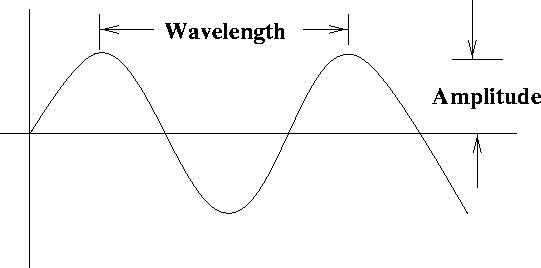Next: Music and Sound Up: Waves Previous: Waves

## Properties of waves

A wave can be defined as follows:It is important to realize that a wave is quite a different object than a particle. A baseball thrown though a window transfers energy from one point to another, but this involves the movement of a material object between two points. A common example of a wave is a wave on the ocean - we know they carry energy, as they cause erosion on the shore, but material (i. e. , water) is not continuously being transferred onto the shore. Another example of a wave is a sound wave, which is vibrations of air molecules which propagate from one place to another. These also carry energy, but do not involve the mass movement of air from one place to another.

A simple type of wave is illustrated below.The main properties of waves are defined below.
• Amplitude: the height of the wave, measured in meters.
• Wavelength: the distance between adjacent crests, measured in meters.
• Period: the time it takes for one complete wave to pass a given point, measured in seconds.
• Frequency: the number of complete waves that pass a point in one second, measured in inverse seconds, or Hertz (Hz).
• Speed: the horizontal speed of a point on a wave as it propagates, measured in meters / second.
Not all of these properties are independent; one has the relations
• Period = 1 / frequency
• Speed = wavelength / period = wavelength x frequencyNext: Music and Sound Up: Waves Previous: Waves
modtech@theory.uwinnipeg.ca
1999-09-29